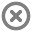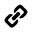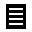Loading...InfoLKS2 Mathematics for Workbook

by Avantis Education UK

158MB

FreeDescription

Practical apps, games and tools designed for use in lower Key Stage Two (Years 3 and 4) to develop maths skills.

Explore the number system, calculation, geometry, statistics, measures and more.

This Lesson Profile has been designed with our latest LearnPad device, the Workbook, in mind. It contains no Flash-based resources.

Contents

• LKS2 Number
•Number Pieces
•Number Line
•Number Rack
•Number Frames
•Place Value Charts
•Concentration: match different representations of number
•Fraction Matcher
•Fraction Game
•Equivalent Fractions BINGO
•Fraction Fling
•Guess the Number
•Place Value Soccer Rounding
•Recipes with Fractions
•Rocket Rounding
•Compare Decimals
•Coconut Ordering
•Range Arranger
•Shark Numbers
•Blast Off
•Match Fractions Decimals and Percentages
•Moving Digits
• LKS2 Geometry
•Cube Nets
•Geometric Solids
•Geoboard
•Pattern Shapes
•Tangrams
•Acute and obtuse angles
•Which 2D Shape?
•Cubes
•Graph Co-ordinates
•Find Places on a Grid Map
•Show the Coordinate
•Make a Walking Track
•Fixpoints
• LKS2 Calculation
•Deep Sea Duel
•Blackboard Times Tables
•TT Xpress
•Broken Calculator
•Maths Vocabulary Cards
•Number Frames
•Number Line
•Number Pieces
•Scales
•Hit the Button
•Balancing Equations
•Product Game (Multiplication)
•Factor Game
•Clear It Multiplication
•Maths BINGO
•Maths Stack
•Arithmetic Tables
•Coconut Multiples
•Connect 4 Factors
•Mental Maths Train
•Maths Chase - Times Tables Games
• LKS2 Statistics
•Create a graph
•Adjustable Spinner
•LearnPad Office Suite
•Interpret Bar Graphs LKS2
•Which Bar Graph is Correct?
•Create Line Graphs
•The Sludger
• LKS2 Measurement
•StopWatch & Timer
•Maths Vocabulary Cards
•Which Metric Unit is Appropriate?
•Length Word Problems
•Find Start and End Times
•Convert Time Units
•Find the Change in Time
•Toy Shop Money
•Coins Game
•Checkout Change Game
•Coconut Ordering
•Measuring in Centimetres
•Two Step Money Problems
•Find the Start Time
• LKS2 Maths Tools
•SimpleMind
•Author
•WorkSpace
•LearnPad Office Suite
•Presenter
•Animator
•Sound Recorder
•Camera
•SketchBook MobileX
•Square Numbers Animation Example
•Daily 10 Mental Maths Challenge